Skip to main content Accessibility help
Home
Hostname: page-component-78dcdb465f-6zfdk Total loading time: 2.15 Render date: 2021-04-17T15:09:11.006Z Has data issue: true Feature Flags: { "shouldUseShareProductTool": true, "shouldUseHypothesis": true, "isUnsiloEnabled": true, "metricsAbstractViews": false, "figures": false, "newCiteModal": false, "newCitedByModal": true }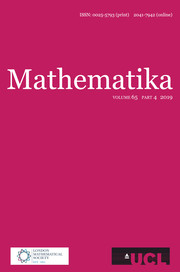Mathematika

# SOLUTIONS TO DIAGONAL CONGRUENCES WITH VARIABLES RESTRICTED TO A BOX

Published online by Cambridge University Press:  03 April 2018

Corresponding
E-mail address:

## Abstract

We prove that for any positive integers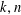$k,n$ with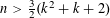$n>\frac{3}{2}(k^{2}+k+2)$ , prime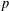$p$ , and integers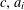$c,a_{i}$ , with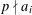$p\nmid a_{i}$ ,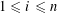$1\leqslant i\leqslant n$ , there exists a solution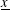$\text{}\underline{x}$ to the congruence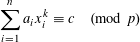$$\begin{eqnarray}\mathop{\sum }_{i=1}^{n}a_{i}x_{i}^{k}\equiv c\hspace{0.6em}({\rm mod}\hspace{0.2em}p)\end{eqnarray}$$
with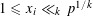$1\leqslant {x_{i}\ll }_{k}p^{1/k}$ ,$1\leqslant i\leqslant n$ . This upper bound is best possible. Refinements are given for smaller$n$ , and for variables restricted to intervals in more general position. In particular, for any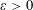$\unicode[STIX]{x1D700}>0$ we give an explicit constant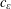$c_{\unicode[STIX]{x1D700}}$ such that if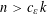$n>c_{\unicode[STIX]{x1D700}}k$ , then there is a solution with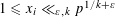$1\leqslant {x_{i}\ll }_{\unicode[STIX]{x1D700},k}p^{1/k+\unicode[STIX]{x1D700}}$ .

## MSC classification

Type
Research Article
Information
Mathematika , 2018 , pp. 430 - 444
Copyright
Copyright © University College London 2018

## Access options

Get access to the full version of this content by using one of the access options below.

## References

Baker, R. C., Small solutions of congruences. Mathematika 30 1983, 164188.CrossRefGoogle Scholar
Baker, R. C., Diophantine Inequalities (London Mathematical Society Monographs), Clarendon Press (Oxford, 1986).Google Scholar
Bourgain, J., Demeter, C. and Guth, L., Proof of the main conjecture in Vinogradov’s mean value theorem for degrees higher than three. Ann. of Math. (2) 184(2) 2016, 633682.Google Scholar
Cilleruelo, J., Garaev, M. Z., Ostafe, A. and Shparlinski, I. E., On the concentration of points of polynomial maps and applications. Math. Z. 272 2012, 825837.CrossRefGoogle Scholar
Cochrane, T., Exponential Sums and the Distributions of Solutions of Congruences (Institute of Mathematics), Academia Sinica (Taipei, Taiwan, 1994), 184.Google Scholar
Cochrane, T., Ostergaard, M. and Spencer, C., Small solutions of diagonal congruences. Funct. Approx. Comment. Math. 56(1) 2017, 3948.Google Scholar
Cochrane, T. and Zheng, Z., Small solutions of the congruence a 1 x 1 2 + a 2 x 2 2 + a 3 x 3 2 + a 4 x 4 2c (mod p). Acta Math. Sinica (N.S.) 14(2) 1998, 175182.CrossRefGoogle Scholar
Dietmann, R., Small solutions of additive cubic congruences. Arch. Math. 75 2000, 195197.CrossRefGoogle Scholar
Ramaswami, V., On the number of positive integers less than x and free of prime divisors greater than x c . Bull. Amer. Math. Soc. 55 1949, 11221127.CrossRefGoogle Scholar
Sárközy, A., On products and shifted products of residues modulo p. Integers 8(2) 2008, A9, 8 pp.Google Scholar
Schmidt, W. M., Small zeros of additive forms in many variables, II. Acta Math. 143(3–4) 1979, 219232.CrossRefGoogle Scholar
Schmidt, W. M., Bounds on exponential sums. Acta Arith. 94(3) 1984, 281297.Google Scholar
Schmidt, W. M., Small solutions of congruences with prime modulus. In Diophantine Analysis, Proc. Number Theory Sect. Aust. Math. Soc. Conv. 1985 (London Mathematical Society Lecture Notes Series 109 ), Cambridge University Press (Cambridge, 1986), 3766.CrossRefGoogle Scholar
Shparlinski, I. E. and Zumalacárregui, A., On the density of zeros of diagonal forms modulo a prime. Preprint, 2017.Google Scholar
Vinogradov, I. M., New estimates for Weyl sums. Dokl. Akad. Nauk SSSR 8 1935, 195198.Google Scholar
Wooley, T. D., New estimates for smooth Weyl sums. J. Lond. Math. Soc. 51 1995, 113.CrossRefGoogle Scholar
Wooley, T. D., Vinogradov’s mean value theorem via efficient congruencing. Ann. of Math. (2) 175 2012, 15751627.CrossRefGoogle Scholar
Wooley, T. D., Vinogradov’s mean value theorem via efficient congruencing, II. Duke Math. J. 162 2013, 673730.CrossRefGoogle Scholar

### Full text views

Full text views reflects PDF downloads, PDFs sent to Google Drive, Dropbox and Kindle and HTML full text views.

Total number of HTML views: 6
Total number of PDF views: 44 *
View data table for this chart

* Views captured on Cambridge Core between 03rd April 2018 - 17th April 2021. This data will be updated every 24 hours.

# Send article to Kindle

To send this article to your Kindle, first ensure no-reply@cambridge.org is added to your Approved Personal Document E-mail List under your Personal Document Settings on the Manage Your Content and Devices page of your Amazon account. Then enter the ‘name’ part of your Kindle email address below. Find out more about sending to your Kindle. Find out more about sending to your Kindle.

Note you can select to send to either the @free.kindle.com or @kindle.com variations. ‘@free.kindle.com’ emails are free but can only be sent to your device when it is connected to wi-fi. ‘@kindle.com’ emails can be delivered even when you are not connected to wi-fi, but note that service fees apply.

Find out more about the Kindle Personal Document Service.

SOLUTIONS TO DIAGONAL CONGRUENCES WITH VARIABLES RESTRICTED TO A BOX
Available formats
×

# Send article to Dropbox

To send this article to your Dropbox account, please select one or more formats and confirm that you agree to abide by our usage policies. If this is the first time you use this feature, you will be asked to authorise Cambridge Core to connect with your <service> account. Find out more about sending content to Dropbox.

SOLUTIONS TO DIAGONAL CONGRUENCES WITH VARIABLES RESTRICTED TO A BOX
Available formats
×

# Send article to Google Drive

To send this article to your Google Drive account, please select one or more formats and confirm that you agree to abide by our usage policies. If this is the first time you use this feature, you will be asked to authorise Cambridge Core to connect with your <service> account. Find out more about sending content to Google Drive.

SOLUTIONS TO DIAGONAL CONGRUENCES WITH VARIABLES RESTRICTED TO A BOX
Available formats
×
×

#### Conflicting interests

Do you have any conflicting interests? *